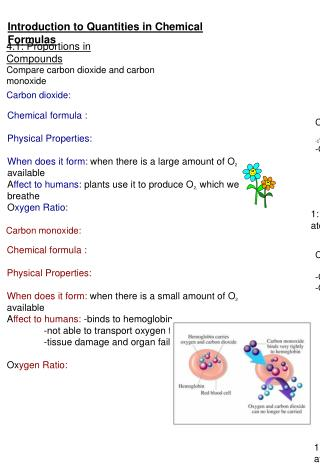DownloadDownload PresentationIntroduction to Quantities in Chemical Formulas

# Introduction to Quantities in Chemical Formulas

Download Presentation## Introduction to Quantities in Chemical Formulas

- - - - - - - - - - - - - - - - - - - - - - - - - - - E N D - - - - - - - - - - - - - - - - - - - - - - - - - - -
##### Presentation Transcript

1. CO2 -Colourless -Odourless CO -Colourless -Odourless Introduction to Quantities in Chemical Formulas 4.1: Proportions in Compounds Compare carbon dioxide and carbon monoxide Carbon dioxide: Chemical formula : Physical Properties: When does it form: when there is a large amount of O2 available Affect to humans: plants use it to produce O2, which we breathe Oxygen Ratio: 1: 2 (1 carbon atom for every 2 oxygen atoms) Carbon monoxide: Chemical formula : Physical Properties: When does it form: when there is a small amount of O2 available Affect to humans: -binds to hemoglobin, -not able to transport oxygen to the body -tissue damage and organ failure Oxygen Ratio: 1: 2 (1 carbon atom for every 2 oxygen atoms) 1: 1 (1 carbon atom for every 1 oxygen atom)

2. Law of Definite Proportions: the proportions of elements in a specific compound, are always the same, no matter how the compound is made. -The properties of a substance will depend on: 1) the elements in the compound 2) the quantity of each element in the compound Ex. 2 : O2 vs. O3 So to analyze chemical reactions quantitatively…. Stoichiometry: the study of the relationships between the quantities of reactants and products involved in chemical reactions

3. H O H O H O H H H O H H O H O O O H H H H O O O H O H H H H H H H H H H Law of Definite Proportions Ex. 1 : Sort the following atoms and determine the compound's chemical formula. Hydrogen Box Oxygen Box # of hydrogens = # of oxygens = : ratio: The compound's chemical formula is: _______________

4. Use the law of definitive proportions to determine the unknown masses. ? hydrogen + chlorine

5. 4.2 Relative Atomic Mass -Relative atomic mass helps us to understand how the mass numbers on the periodic table were found -When John Dalton came up with the Billiard Ball model, he also determined a way to express the mass of atoms -What was the billiard ball model and what did it tell us about the mass of atoms?

6. - LDP : each time calcium oxide is formed; the proportions of calcium and oxygen that combine to form calcium oxide will always be the same \1atom of calcium is 2.5 times heavier than 1 atom of oxygen -Using this information and the law of definite proportions, Dalton was able to express the masses of atoms RELATIVE TO EACH OTHER! -For example, if we obtain the following results from the reaction of calcium and oxygen to form calcium oxide: calcium + oxygen calcium oxide 40g 16g 56g

7. -Carbon is a very common element that is found in almost every naturally occurring compound. - if we use C-12 the atomic masses of nearly all other elements are very close to whole number, -C-12 makes up out 98% of all naturally occurring carbon -Now, Dalton used hydrogen first because it was the lightest element and gave it a mass of 1. -he compared all the other element to this value For ex. : when Dalton looked at water, he saw thta 1 g of hydrogen combined with 8 g oxygen -so he gave oxygen a mass of 8 -this was a mistake since 2 atoms of oxygen combines with one atom of hydrogen -So oxygen must have a relative atomic mass of 16 So what do we mean by relative atomic mass Relative atomic mass: mass of an element that would react with a fixed mass of a standard element -we are currently using carbon -12 as our standard element Why?

8. Units, Units, Units! -Remember IUPAC and SI units, the proper units for the mass of an atom are atomic mass units or "u" Atomic mass unit: 1 u is equal to 1/12th the mass of a carbon-12 atom and just to put this into perspective: 1 u = 1.66´ 10-27 kg (thats really tiny!) Ex.1 - What is the mass of 1 carbon-12 atom? Use atomic mass units. Ex.2 - If a titanium atoms weighs 4 times a carbon-12 atom, calculate the atomic mass of titanium in u. Bonus: Express this in kg

9. Homework Pg.162 #1 Pg.164 # 1,3,4